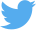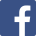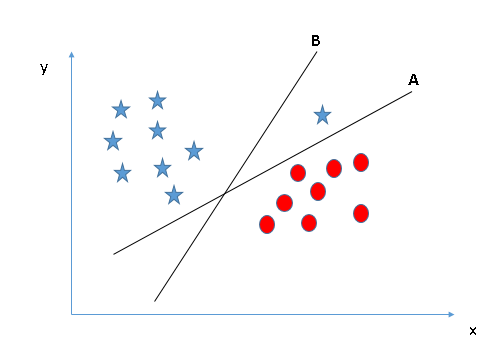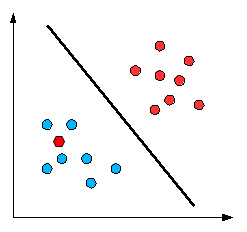Learn
Turtle
Support Vector MachineLearn
TurtleSupport Vector MachineSupport Vector Machine

# Hello Learner!!!!,

We are going to deal with Support Vector Machine in Machine Learning. We will use Python to code.

## Let us see Table of Content -

a) What is Support Vector Machine?

b) How does SVM work?

c) Implementation of SVM in Python

## What is Support Vector Machine (SVM)??

In machine learning, support-vector machines (SVMs, also support-vector networks) are supervised learning models with associated learning algorithms that analyze data used for classification and regression analysis. It is mainly used for classification problem. In the SVM algorithm, we plot each data item as a point in n-dimensional space (where n is number of features you have) with the value of each feature being the value of a particular coordinate. Then, we perform classification by finding the hyper-plane that differentiates the two classes very well (look at the below snapshot).Support vectors are data points that are closer to the hyperplane and influence the position and orientation of the hyperplane. Using these support vectors, we have to maximize the margin of the classifier. These are the points that help us build our SVM.

## How does SVM Work?

From the above discussion, it is clear that our aim to find a hyper-plane segregating the two classes.

So, Let’s discuss the same with some scenario:

1) From the image it is clear hyper-plane B is the correct choice.2) In the below image plane A,B and C seems right then what should we do??We will maximize the distances between nearest data point (either class) and hyper-plane which will help us to decide the right hyper-plane and this distance is called as Margin.

We can see margin for C is grater then B and A so C is more accurate. If we select a hyper-plane having low margin then there is high chance of miss-classification. Selecting a hyper-plane with low margin will create high chance of miss-classification

3) In the below image it seems B is more accurate as it has high margin but A is more favorable because B gas a classification error and A has classified all correctly.4) In the below image we cant find any plane which can separate all data points but no need to worry SVM can ignore few points to get the required hyper-plane.5) In the below image we can’t find linear hyper-plane, this problem is handled by SVM by creating extra features. Considering about above case if we can apply z = x^2 + y^2 then it will look likeExtra features are not added by us, the SVM algorithm has a technique called the kernel trick which is used for addition of extra features. The SVM kernel is a function that takes low dimensional input space and transforms it to a higher dimensional space i.e. it converts not separable problem to separable problem. It is mostly useful in non-linear separation problem. Simply put, it does some extremely complex data transformations, then finds out the process to separate the data based on the labels or outputs you’ve defined.

We will not discuss math’s behind SVM. Comment in the comment section if you want to know math’s behind. I will try to make an article on that also.

## How to Implement SVM in Python???

``````from sklearn.svm import SVC
classifier = SVC(kernel = 'linear', random_state = 0)
classifier.fit(X_train, y_train)

# Predicting the Test set results
y_pred = classifier.predict(X_test)

``````

That’s it….

Training Set -Test Set -This is the extra code required, Yesss some more lines of code is required to visualize But this is the main code to get complete code and data please visit here.

• Performance is better when there is clear margin of separation. • Effective in high dimensional spaces. • Effective in cases where the number of dimensions is greater than the number of samples. • It is memory efficient as it uses a subset of training points in the decision function (called support vectors).